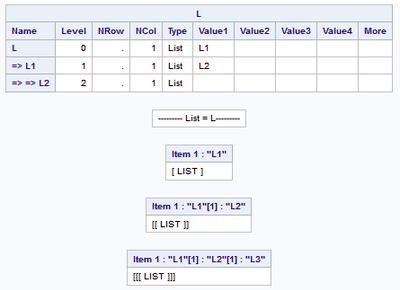## Why don't Struct(L) and ListPrint(L) display all of the items of a list?

Here is an example of a list that has four levels of nesting:

``````proc iml ; package load listutil ;
L = [ #'L1' = [ #'L2' = [ #'L3' = [ #'L4' = []]]]] ;
call struct( L ) ;
call listprint( L ) ;
quit ;
``````

When I look at the results, I seeand no indication of sublist L4. Sublist L3 is mentioned in the Listprint() call and apocryphically in the Struct() call by the fact that name L2 is a pointer to a list, but what happened to sublist L4?

How can I see all of the objects of a list and the complete structure of a list?

1 ACCEPTED SOLUTION

Accepted Solutions

## Re: Why don't Struct(L) and ListPrint(L) display all of the items of a list?

In SAS 9, the STRUCT and LISTPRINT functions are implemented as SAS/IML modules that are defined in the ListUtil package. They are only implemented to support up to three levels of nesting.  You can run STRUCT on sublists to see additional structures, such as

L111 = L\$1\$1\$1;

RUN STRUCT(L111);

In SAS Viya, the iml action and the IML procedure support the STRUCT subroutine and the LISTPRINT subroutine as built-in functions. You do not need to load a package. In Viya, these functions display the complete structure of lists to arbitrary depths of nesting.

5 REPLIES 5

## Re: Why don't Struct(L) and ListPrint(L) display all of the items of a list?

In SAS 9, the STRUCT and LISTPRINT functions are implemented as SAS/IML modules that are defined in the ListUtil package. They are only implemented to support up to three levels of nesting.  You can run STRUCT on sublists to see additional structures, such as

L111 = L\$1\$1\$1;

RUN STRUCT(L111);

In SAS Viya, the iml action and the IML procedure support the STRUCT subroutine and the LISTPRINT subroutine as built-in functions. You do not need to load a package. In Viya, these functions display the complete structure of lists to arbitrary depths of nesting.

## Re: Why don't Struct(L) and ListPrint(L) display all of the items of a list?

Is the source code for ListPrint() and struct() available so that I might
customize the modules to expand their capabilities? It's hard to constantly
specify subitems of a list to display the entire list.

## Re: Why don't Struct(L) and ListPrint(L) display all of the items of a list?

You can see the source code by running:

``package info listutil;``

This will tell you the name of the directory where the package is stored and the IML code should be in the sub-directory called 'source'.  There is no need to rewrite ListPrint, you are welcome to use my version below.

``````proc iml;

start L_Print( L, UseNames=0, labl=("--------- List = " + parentname("L") + "---------"),
maxdepth=30);
if type(L) ^= 'L' then return;
/* i indexes items at each depth, n is the list length at each depth. */
i = j(1, maxdepth, 0);
n = j(1, maxdepth, 0);
vnam = 'nam1':('nam'+char(maxdepth));
nstr = j(1, maxdepth, blankstr(40));
d = 1;  /* the current depth in the list */
print labl[L=""];
nam1 = ListGetAllNames( L );
n = ListLen( L );
if ncol(nam1)=0 then nam1 = char(1:n);
do until( (d = 0) | (d > maxdepth) );
i[d] = i[d] + 1;
if i[d] <= n[d] then do;
x = ListGetSubItem( L, i[1:d] );
nstr[d] = value(vnam[d])[i[d]];
if nstr[d] = ' ' then nstr[d] = char(i[d]);
if UseNames
then msg = cats(parentname('L'),rowcatc( "['" + nstr[,1:d] + "']"));
else msg = cats(parentname('L'),rowcatc( '[' + char(i[,1:d]) + ']'));
if type(x)='U'
then print ('Undefined') [label=msg];
else if type(x)='C' | type(x)='N' then do;
if ncol(x)=1 then x = t(x);
print x[label=msg];
end; else if type(x)='T' then
call TablePrint(x) label=msg;
else if type(x)='L' then do;
print (cshape('[',1,1,d) + ' LIST ' + cshape(']',1,1,d)) [label=msg];
d = d + 1;
n[d] = ListLen(x);
tn = ListGetAllNames(x);
if ncol(tn)=0 then tn = char(1:n[d]);
call ValSet(vnam[d], tn);
end;
end; else do;
i[d] = 0;
d = d - 1;  /* move up the list one level */
end;
end;
finish;

L = [ #'L1' = [ #'L2' = [ #'L3' = [ #'L4' = []]]]] ;
call L_print( L, 1 ) ;

quit;``````

## Re: Why don't Struct(L) and ListPrint(L) display all of the items of a list?

Ian, thank you very much for sharing your code with the SAS/IML community.
Your L_Print module is a model of clarity in form and function and I will benefit from learning how lists are structured and manipulated by studying your module.

## Re: Why don't Struct(L) and ListPrint(L) display all of the items of a list?

Thanks for your kind words.  I should make it clear that I did exactly what you proposed to do above, and started from the code in the package.  So I would like to acknowledge Rick for writing the original ListPrint - I definitely learned a few things from his code!

From The DO Loop